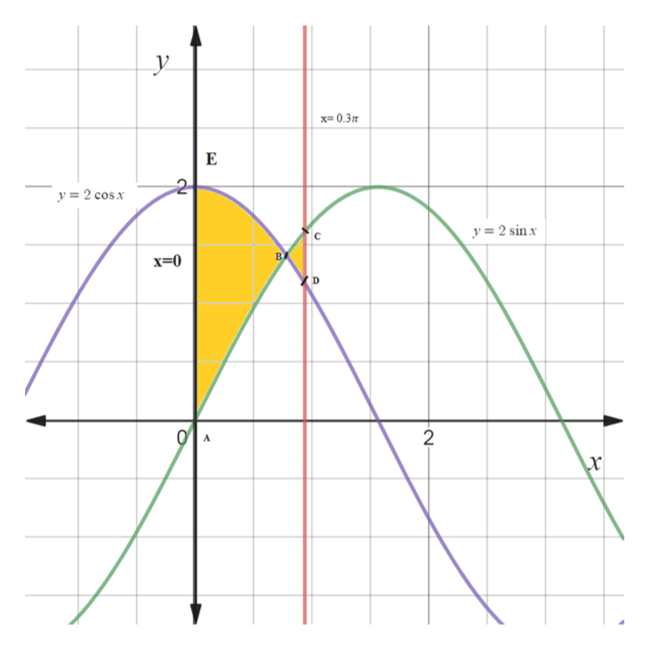# Find the area of the region enclosed between y=2sin(x) and y=2cos(x) from x=0 to x=0.3π. Hint: Notice that this region consists of two parts

Question
368 views

Find the area of the region enclosed between y=2sin(x) and y=2cos(x) from x=0 to x=0.3π. Hint: Notice that this region consists of two parts

check_circle

Step 1

To find the area enclosed between y= 2sin(x) and y= 2cos(x) from x=0 to x= 0.3

Step 2

Given information:

Step 3

Graph of the curves i...help_outlineImage Transcriptioncloseхн 0.Зл y = 2 cos x y = 2 sin x в х-0 fullscreen

### Want to see the full answer?

See Solution

#### Want to see this answer and more?

Solutions are written by subject experts who are available 24/7. Questions are typically answered within 1 hour.*

See Solution
*Response times may vary by subject and question.
Tagged in
MathCalculus

### Integration